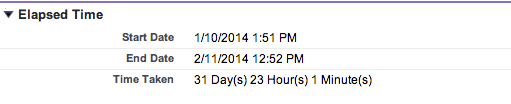Here is the formula to calculate the difference between two dates in Salesforce. The difference is returned in Days, Hours & Minutes. Works like a charm.

```TEXT( FLOOR(End_Date__c - Start_Date__c) ) & " Day(s) " & TEXT( ROUND(MOD((End_Date__c - Start_Date__c)*24,24),0) ) &" Hour(s) " & TEXT( ROUND(MOD((End_Date__c - Start_Date__c)*1440,60),0) ) &" Minute(s) "```